# Convert Matrix to Vector in R

In this article, we are going to convert the given matrix into the vector in R programming language.

## Conversion of the matrix to vector by row

### Method 1: Using c() function

Simply passing the name of the matrix will do the job.

Syntax:

c(matrix_name)

Where matrix_name is the name of the input matrix

Example 1:

## R

 `# create a matrix with 12 elements ` `# with 4 rows and 3 columns` `matrix=``matrix``(1:12,nrow=4,ncol=3)` `print``(matrix)`   `# convert matrix to vector using c()` `# function` `a=``c``(matrix)`   `print``(a)`

Output: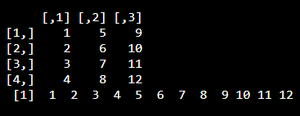Example 2:

## R

 `# create a matrix with 16 elements ` `# with 4 rows and 4 columns` `matrix=``matrix``(1:16,nrow=4,ncol=4)` `print``(matrix)`   `# convert matrix to vector using ` `# c() function` `a=``c``(matrix)`   `print``(a)`

Output: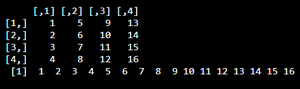### Method 2: Using as.vector() function

This function is used to convert matrix to vector so again simply passing the matrix name is enough.

Syntax:

as.vector(matrix)

Example:

## R

 `# create a matrix with 12 elements` `# with 4 rows and 3 columns` `matrix=``matrix``(1:12,nrow=4,ncol=3)` `print``(matrix)`   `# convert matrix to vector using` `# as.vector() function` `a=``as.vector``(matrix)`   `print``(a)`

Output: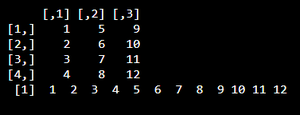Example 2:

## R

 `# create a matrix with 16 elements with 4 rows and 4 columns` `matrix=``matrix``(1:16,nrow=4,ncol=4)` `print``(matrix)`   `# convert matrix to vector using as.vector() function` `a=``as.vector``(matrix)`   `print``(a)`

Output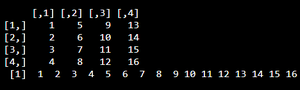## Conversion of the matrix to vector by column

### Method 1: Using c() function along with t() function

t() function is used to transpose the given matrix. It will transpose rows as columns and columns as rows.

Syntax:

t(matrix)

where the matrix is the input matrix

After applying t() we can apply c() and as.vector() functions to convert the matrix to vector

Syntax:

c(t(matrix))

Example 1:

## R

 `# create a matrix with 12 elements ` `# with 4 rows and 3 columns` `matrix=``matrix``(1:12,nrow=4,ncol=3)` `print``(matrix)`   `# convert matrix to vector using ` `# c() function along with t()` `a=``c``(``t``(matrix))`   `print``(a)`

Output: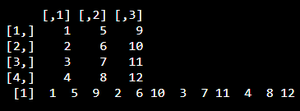Example 2:

## R

 `# create a matrix with 12 elements` `# with 2 rows and 6 columns` `matrix=``matrix``(1:12,nrow=2,ncol=6)` `print``(matrix)`   `# convert matrix to vector using ` `# c() function along with t()` `a=``c``(``t``(matrix))`   `print``(a)`

Output: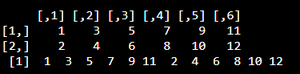### Method 2: Using as.vector() function along with t() function

The work of t() is same as above. After the transpose has been taken, the matrix is converted to vector using as.vector().

Syntax:

as.vector(t(matrix))

Example

## R

 `# create a matrix with 12 elements` `# with 2 rows and 6 columns` `matrix=``matrix``(1:12,nrow=2,ncol=6)` `print``(matrix)`   `# convert matrix to vector using ` `# as.vector() function along with t()` `a=``as.vector``(``t``(matrix))`   `print``(a)`

Output:Example 2:

## R

 `# create a matrix with 4 elements` `# with 2 rows and 2 columns` `matrix=``matrix``(1:4,nrow=2,ncol=2)` `print``(matrix)`   `# convert matrix to vector using ` `# as.vector() function along with t()` `a=``as.vector``(``t``(matrix))`   `print``(a)`

Output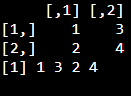Whether you're preparing for your first job interview or aiming to upskill in this ever-evolving tech landscape, GeeksforGeeks Courses are your key to success. We provide top-quality content at affordable prices, all geared towards accelerating your growth in a time-bound manner. Join the millions we've already empowered, and we're here to do the same for you. Don't miss out - check it out now!

Previous
Next